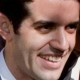# Convert from latitude and longitude to point/pixel in Google Maps V3

Hi all!

Migrating eattopia from google maps v2 to v3 in GWT we have had lots of problems. First is all those api changes. And… although v3 is much more complete it lacks some basic methods in V2. Our main headache has been converting from lat/lon to a point (pixel), just to know where to place the balloons in the map. At the, after investigating, we implemented the following method:

public static Point convertFromLatLngToPoint(LatLng latlng,GoogleMap map,Element elementContainingMap)
{
double worldMapWidth = 256*map.getZoom();

double mapWidth = elementContainingMap.getClientWidth();
double mapHeight = elementContainingMap.getClientHeight();

double mapLonRight = map.getBounds().getNorthEast().lng();
double mapLonLeft = map.getBounds().getSouthWest().lng();
double mapLonDelta= mapLonRight-mapLonLeft;

double mapLatBottom = map.getBounds().getSouthWest().lat();
double mapLatBottomDegree = mapLatBottom * Math.PI / 180;

double longitude = latlng.lng();
double latitude = latlng.lat();

double x = (longitude – mapLonLeft) * (mapWidth / mapLonDelta);

latitude = latitude * Math.PI / 180;
worldMapWidth = ((mapWidth / mapLonDelta) * 360) / (2 * Math.PI);
double mapOffsetY = (worldMapWidth / 2 * Math.log((1 + Math.sin(mapLatBottomDegree)) / (1 – Math.sin(mapLatBottomDegree))));
double y = mapHeight – ((worldMapWidth / 2 * Math.log((1 + Math.sin(latitude)) / (1 – Math.sin(latitude)))) – mapOffsetY);

Point result = Point.create(x, y);

return result;
}

1.1.